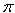SEARCH HOMEMath Central Quandaries & QueriesQuestion from Gerry, a student: How do I figure the percentage between a small circle and a large circleHi Gerry,

I am going to show you how to calculate the percentage increase as you go from one circle to a larger circle. First however I need to decide what aspect of the circles you want to compare. Is it the areas or perhaps the radii, or the circumferences?

I am going to look at two circles, one of radius 4 inches and the other of radius 5 inches.

What is the percentage increase in the radii as you go from the smaller circle to the larger circle?

Percentage increase is (the larger number - the smaller number)/(the smaller number)100. Hence for these circles the percentage increase in the radii is

(5 - 4)/4100 = 25%.

Hence there is a 25% increase in the radius going from a circle of radius 4 inches to a circle of radius 5 inches.

What is the percentage increase in the area as you go from the smaller circle to the larger circle?

The area of a circle isr2 where r is the radius and hence the percentage increase in the area is

(52 -42)/(42)100 = (25 - 16)/16100 = 56%

Hence there is a 56% increase in the area going from a circle of radius 4 inches to a circle of radius 5 inches.

I hope this helps,
PennyMath Central is supported by the University of Regina and The Pacific Institute for the Mathematical Sciences.